Geometry 8-6 Law of Cosines
starstarstarstarstarstarstarstarstarstar
by Matthew Richardson
| 20 Questions
Note from the author:
A complete formative lesson with embedded slideshow, mini lecture screencasts, checks for understanding, practice items, mixed review, and reflection. I create these assignments to supplement each lesson of Pearson's Common Core Edition Algebra 1, Algebra 2, and Geometry courses. See also mathquest.net and twitter.com/mathquestEDU.
The outlined content above was added from outside of Formative.
The outlined content above was added from outside of Formative.
Sovle It! In the diagram below, △ABC is an acute triangle. Use what you know about right triangle trigonometry to write an expression for the area of the shaded region that uses a, b, and c.1
10 pts
Solve It! Step 1: Consider the smaller right triangle. Write an equation relating its unknown leg length (also the width of the orange rectangle) with b and C.

2
10 pts
Solve It! Step 2: Solve the equation from the previous item for x.
x = b cos(C)
x = C cos(b)
x = b sin(C)
x = tan(bC)
We can see that the area of the shaded region = ax, but the instructions are to write an expression for the area of the shaded region that uses a, b, and c.
3
10 pts
Solve It! Step 3: Use the Substitution Property of Equality and the Commutative Property of Multiplication to find the equation that satisfies the Solve It!.
Area of shaded rectangle = aC cos(b)
Area of shaded rectangle = ab cos(C)
Area of shaded rectangle = aC sin(b)
Area of shaded rectangle = ab sin(C)The outlined content above was added from outside of Formative.
The outlined content above was added from outside of Formative.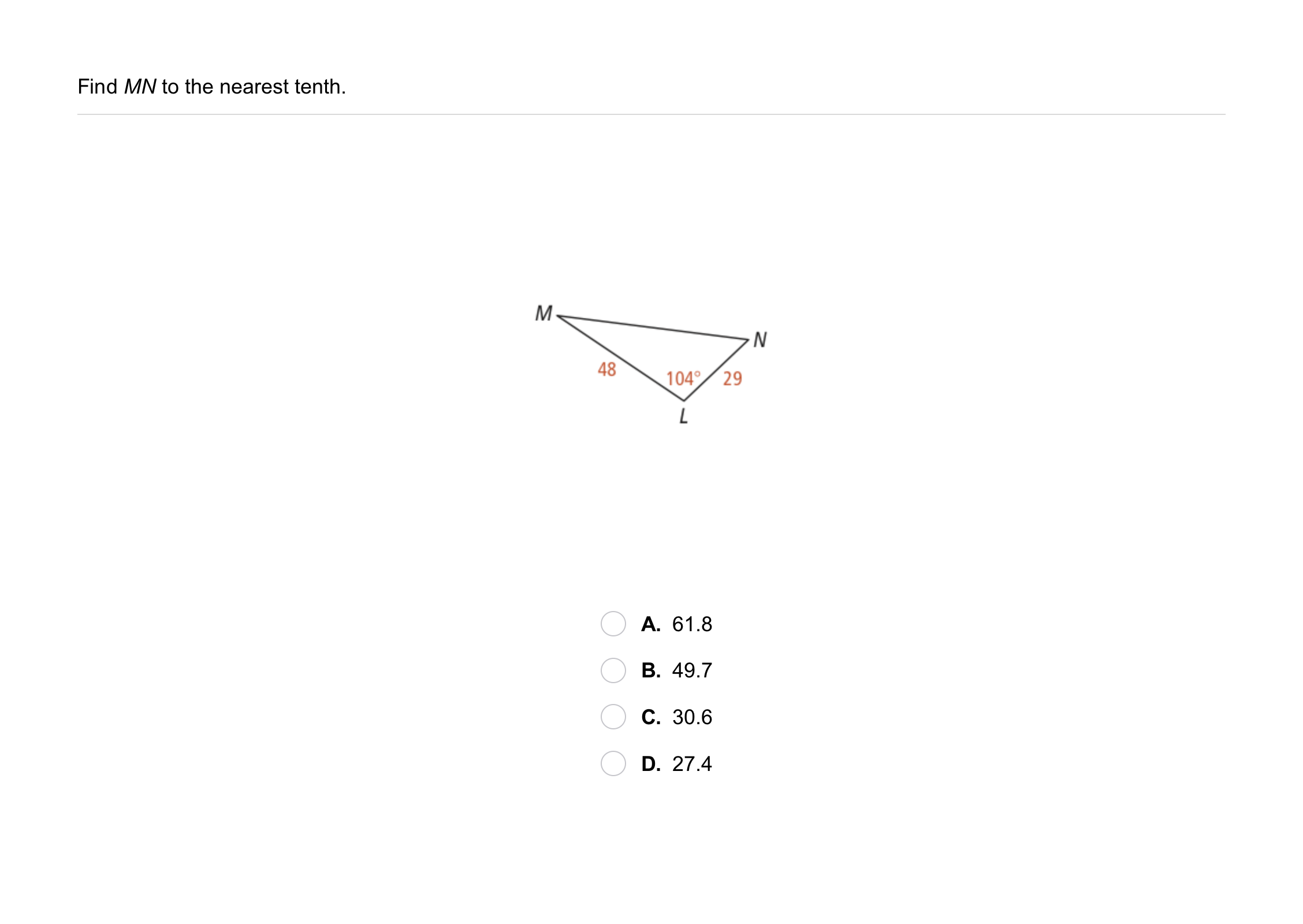4
4
10 pts
Problem 1 Got It?
A
B
C
D
The outlined content above was added from outside of Formative.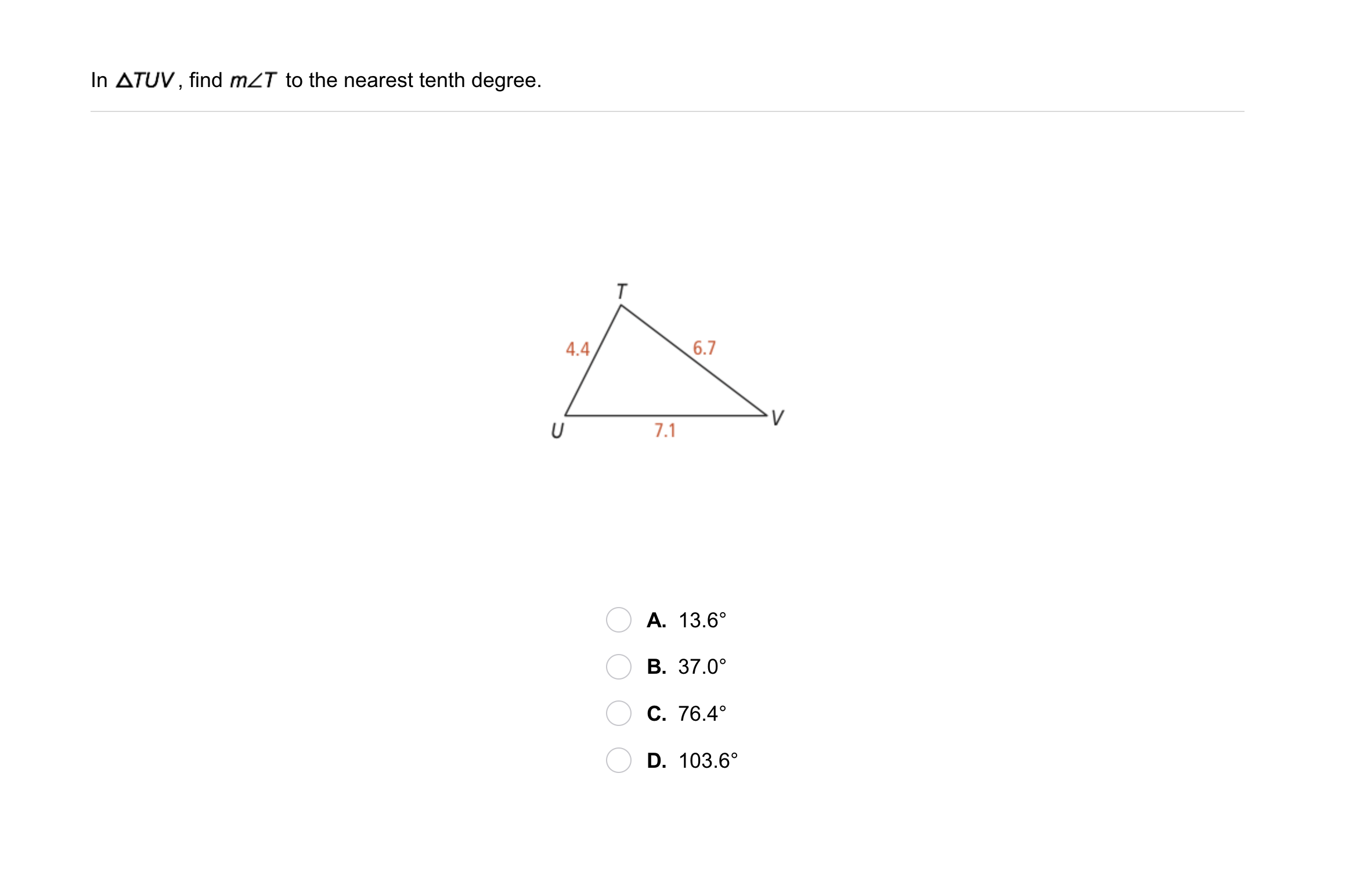5
5
10 pts
Problem 2 Got It?
A
B
C
D
The outlined content above was added from outside of Formative.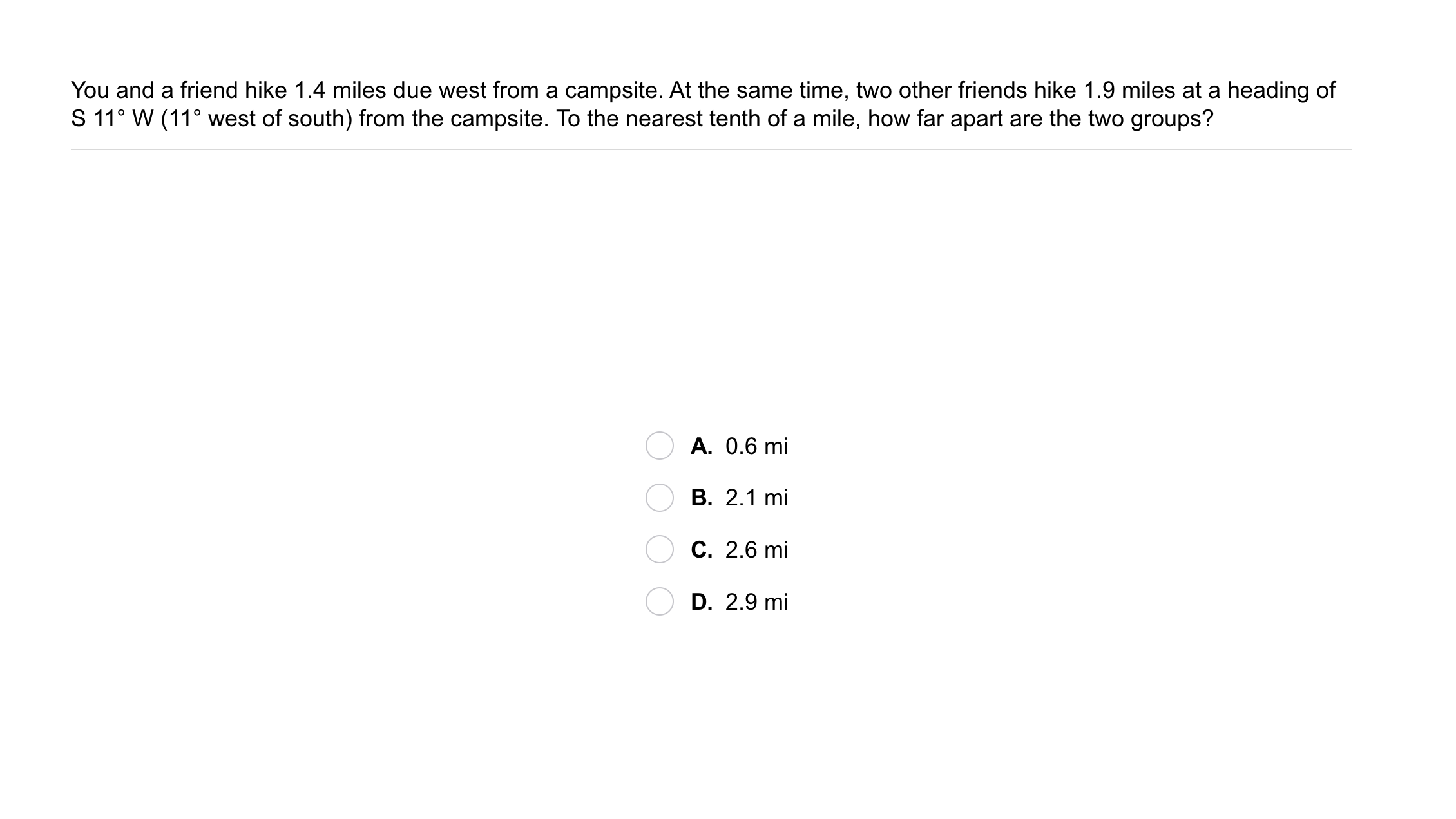6
6
10 pts
Problem 3 Got It?
A
B
C
DThe outlined content above was added from outside of Formative.7
7
10 pts
A
B
C
D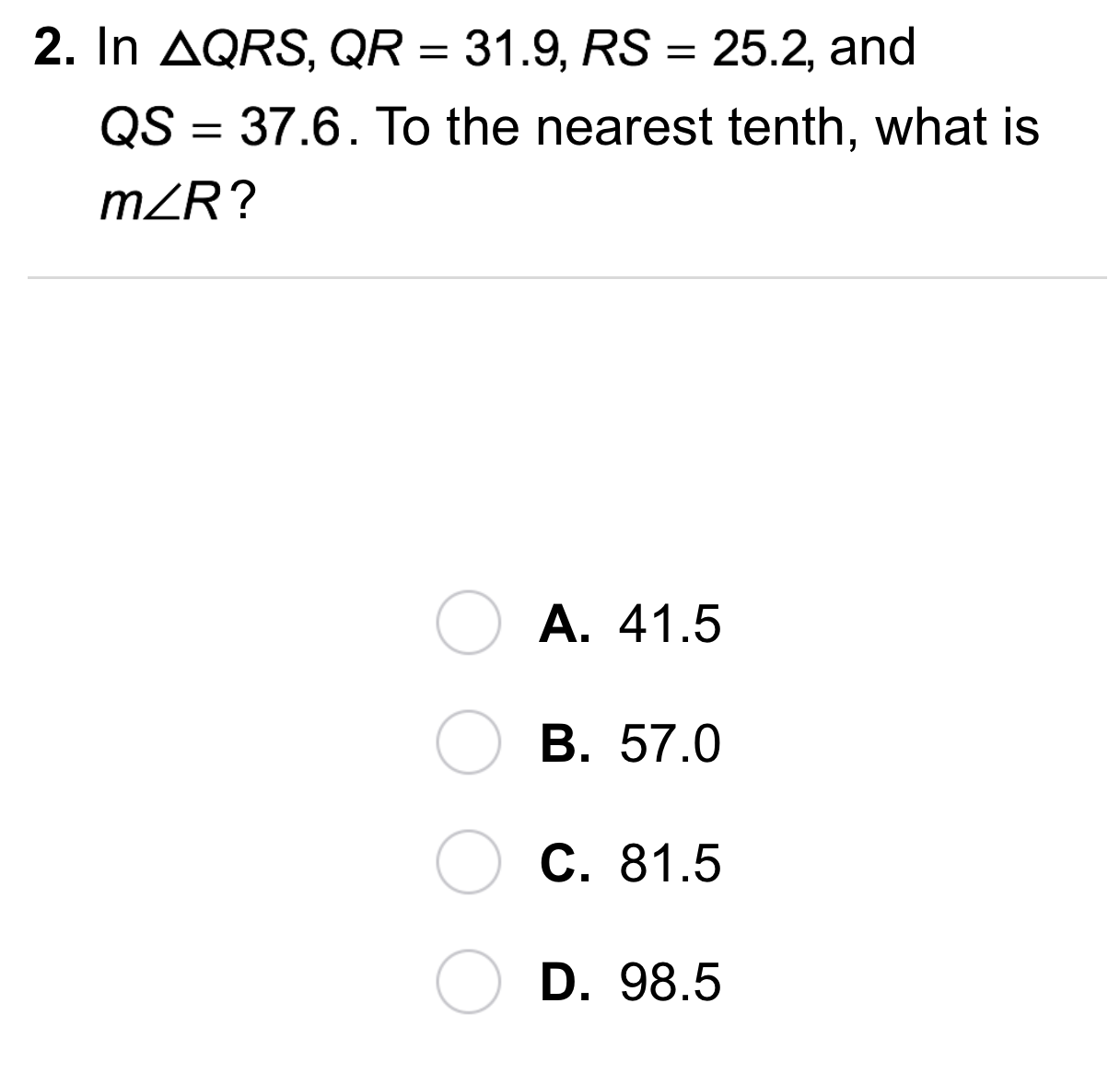8
8
10 pts
A
B
C
D9
9
10 pts
A
B
C
D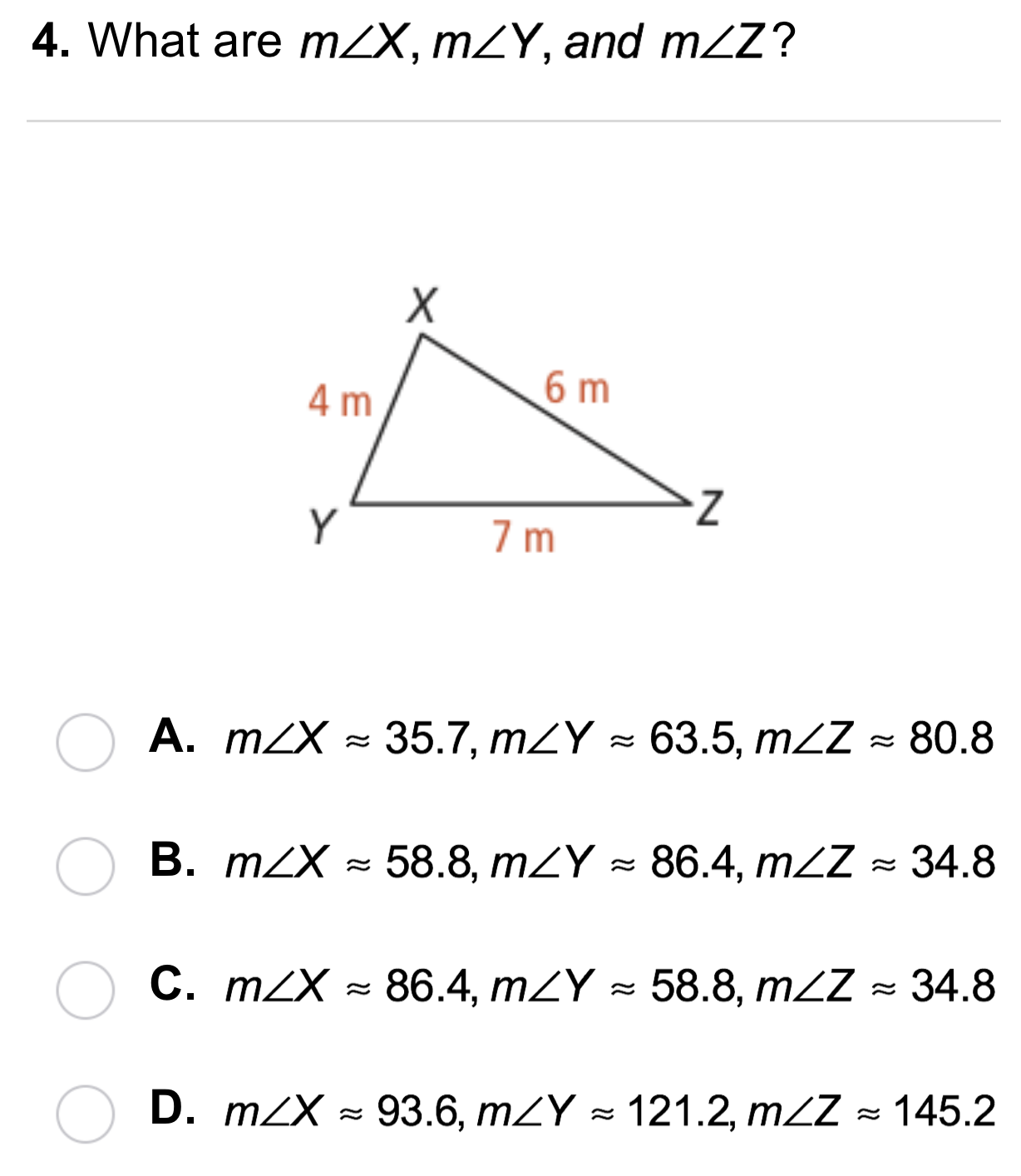10
10
10 pts
A
B
C
DThe outlined content above was added from outside of Formative.11
11
10 pts
Error Analysis: In △ABC, AC = 15 ft, BC = 12 ft, and m∠C = 32. A student solved for c for a = 12 ft, b = 15 ft, and m∠C = 32. What was the error?13
10 pts
Review Lesson 8-4: The first leg of a bike race is 6 km due east. For the second leg of the race, the riders turn northwest and ride 9 km. The final leg of the race runs at a 40° angle back to the second leg and brings the racers back to the starting point. To the nearest tenth, at what angle measure does the third leg of the race meet the first leg? Enter only a number.
14
5 pts
Review Lesson 6-7: Is the quadrilateral with vertices A(-1, -5), B(6, -5), C(9, 3), and D(2, 3) a parallelogram?
Yes
No16
10 pts
Review Lesson 4-1: In the diagram above, △ABC ≅ △EFG. Use this information to match congruent parts from the triangles.
• segment AB
• segment FG
• ∠E
• ∠C
• segment EG
• segment BC
• ∠G
• segment EF
• segment AC
• ∠A17
10 pts
Vocabulary Review: Match the parts from the triangle above in the left column with the descriptions in the right column. Not all parts of the triangle will be used.
• a
• b
• c
• A
• B
• C
• Sides adjacent to angle A
• Side opposite angle B
• Sides adjacent to angle C
18
10 pts
Vocabulary Review: Classify each angle as acute, obtuse, or right.
• 45°
• 100°
• 90°
• 61°
• 110°
• 179°
• Acute
• Obtuse
• Right19
10 pts
Use Your Vocabulary: Identify each ratio as representing sine, cosine, tangent, or none of these.
• opposite/hypotenuse